Electron. J. Diff. Eqns., Vol. 2001(2001), No. 35, pp. 1-15.

### Some observations on the first eigenvalue of the p-Laplacian and its connections with asymmetry Tilak Bhattacharya

Abstract:
In this work, we present a lower bound for the first eigenvalue of the p-Laplacian on bounded domains in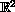. Let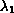be the first eigenvalue and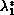be the first eigenvalue for the ball of the same volume. Then we show that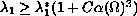, for some constant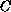, whereis the asymmetry of the domain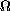. This provides a lower bound sharper than the bound in Faber-Krahn inequality.

Submitted September 3, 2000. Published May 16, 2001.
Math Subject Classifications: 35J60, 35P30.
Key Words: Asymmetry, De Giorgi perimeter, p-Laplacian, first eigenvalue, Talenti's inequality.

Show me the PDF file (261K), TEX file, and other files for this article.Tilak Bhattacharya Indian Statistical Institute 7, S.J.S. Sansanwal Marg New Delhi 110 016 India e-mail: tlk@isid.isid.ac.in